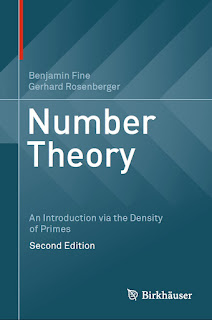THEORETICAL MATHEMATICS

# Number Theory An Introduction via the Density of Primes 2nd Edition Free DownloadNow in its second edition, this textbook provides an introduction and overview of number theory based on the density and properties of the prime numbers. This unique approach offers both a firm background in the standard material of number theory, as well as an overview of the entire discipline. All of the essential topics are covered, such as the fundamental theorem of arithmetic, theory of congruences, quadratic reciprocity, arithmetic functions, and the distribution of primes. New in this edition are coverage of p-adic numbers, Hensel’s lemma, multiple zeta-values, and elliptic curve methods in primality testing.